# STM simulations of tunneling anisotropic magneto resistance (TAMR)¶

Version: S-2021.06

In this tutorial you will learn how to use the LocalDensityOfStates analysis object for simulating STM measurements of surfaces.

In particular we will be studying the tunneling anisotropic magneto resistance (TAMR) which is a measure of the difference in STM current and conductance depending on the magnetization direction of the substrate.## Introduction¶

Scanning tunneling spectroscopy is an experimental technique which uses a scanning tunneling microscope (STM) to probe the local density of electronic states (LDOS) of surfaces and materials on surfaces at the atomic scale. A scanning tunneling spectrum is obtained by placing the STM tip above a particular place on the sample. With the height of the tip fixed, the electron tunneling current is measured as a function of electron energy by varying the voltage between the tip and the sample. The conductance, dI/dV, obtained from such a measurement is proportional to the local electron density of states (LDOS) at the position of the tip.

### TAMR¶

The resistance (inverse of conductance) of a tunneling junction involving just a single ferromagnetic layer may depend on the magnetization direction relative to the crystallographic axes. This effect is called tunneling anisotropic magnetoresistance (TAMR) and has been observed in several STM experiments [NeelSchroderR+13], [SchonebergFH+18]. The TAMR is caused by spin-orbit coupling, which results in an anisotropy of the density of states in and above the surface layer. The TAMR is potentially interesting for spintronics applications as it requires only one magnetic layer as opposed to traditional magnetic tunneling junctions involving both a reference and free magnetic layer.

This tutorial is inspired by the experimental and theoretical works in [NeelSchroderR+13], [SchonebergFH+18] which studies the TAMR of an iron bilayer on a W(110) surface, 2LFe/W(110), as schematically illustrated Fig. 1 below.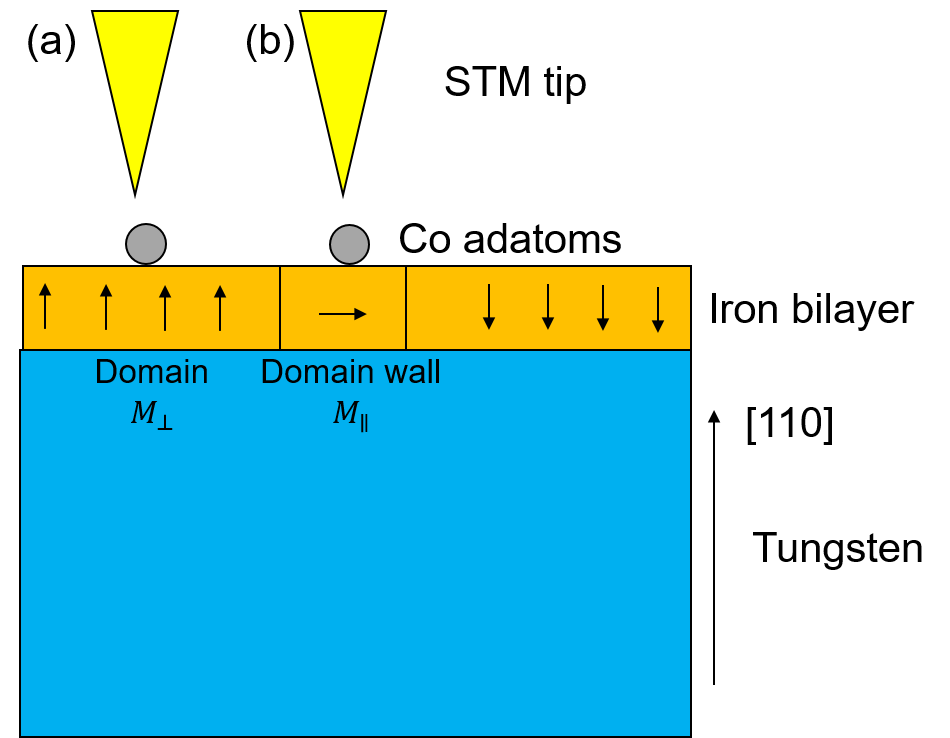Fig. 1 Schematic illustration of the experiments. An iron bilayer is deposited on W(110). The Fe bilayer forms magnetic domains with the magnetization perpendicular to the interface. The magnetic domains are separated by domain walls in which the magnetization is parallel with the interface plane. By positioning the STM tip either over the domain (a) or over the domain wall (b) it is possible to measure the TAMR effect.

This surface can be prepared in such a way that finite domains of out of plane magnetizations are formed. Within the domain wall the magnetization will be in-plane. By measuring with STM the conductance over a domain you get the out of plane component $$(dI/dV)_\perp$$ while the same measurement over a domain wall you get the in-plane component $$(dI/dV)_\parallel$$. The TAMR is then found as

$TAMR = \frac{(dI/dV)_\perp - (dI/dV)_\parallel}{(dI/dV)_\perp}$

The main experimental results from [NeelSchroderR+13] are shown in the figure below. Panel (a) shows the measured $$dI/dV$$ over a Co adatom on a 2LFe/W(110) surface for both in- and out of plane magnetization and panel (b) shows the TAMR as function of sample voltage.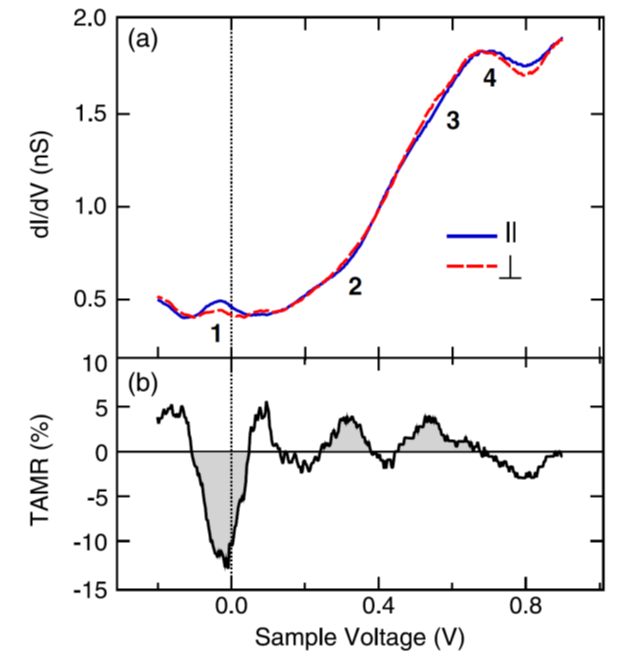Fig. 2 Experimental results. (a) $$dI/dV$$ spectra obtained on Co adatoms on domains (red dashed line) and on domain walls (blue solid line) of an Fe double-layer film on W(110). (b) TAMR determined from the spectra in (a). From [NeelSchroderR+13].

### Tersoff-Hamann approximation of simulated STM¶

The LocalDensityOfStates can be used to simulate scanning tunneling microscopy (STM) images within the Tersoff-Hamann approximation [TH85] assuming that the tip wave function has s-orbital character and is non-magnetic. When simulating an STM image, the energy can be interpreted as the bias difference applied between the tip and the surface and the LocalDensityOfStates represents the STM $$dI/dV$$ measurement.

Since the local density of states $$n(E,r) = A \frac{dI}{dV}|_{V=V_{s}}$$ with $$E=e\cdot V_{s}$$ where $$V_{s}$$ is the sample voltage, we can simulate the TAMR as

(1)$TAMR = \frac{n_\perp(E,r) - n_\parallel(E,r)}{n_\perp(E,r)}$

Likewise, the current measured in an STM with an applied bias of $$V_{s}$$ and with the tip at zero bias can be approximated as the partial electron density originating from eigenstates in the energy interval $$[0; e\cdot V_{s}]$$.

## Setting up the 2LFe/W(110) structure¶

We will start by setting up the structure for the calculations.

### Structure¶

Open the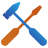Builder and

• Add a Wolfram/Tungsten bulk configuration from the database (search for Wolfram).
• Make a (110) surface using the Surface (Cleave) under Builders in the right column.
• On the first page set h=1, k=1, l=0 . Press Next.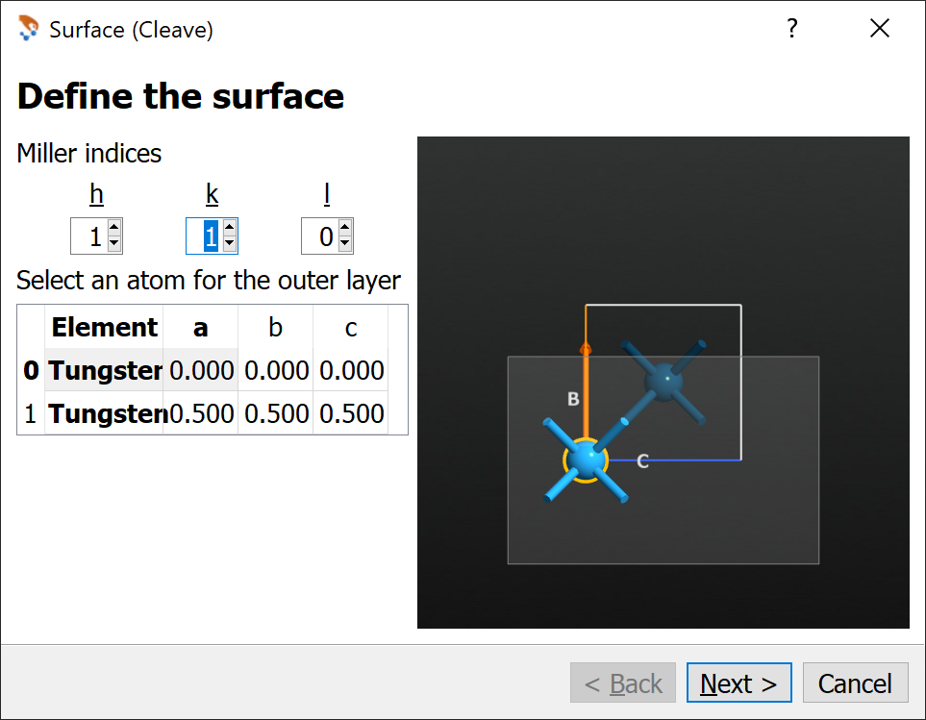• On the second page simply press Next using all default values.
• On the third page apply the changes (see image below)
• From the combo box choose Non-periodic and normal (slab)
• Set the Top and Bottom vacuum to 5 layers
• Set the Thickness to 10 layers
• Press Finish.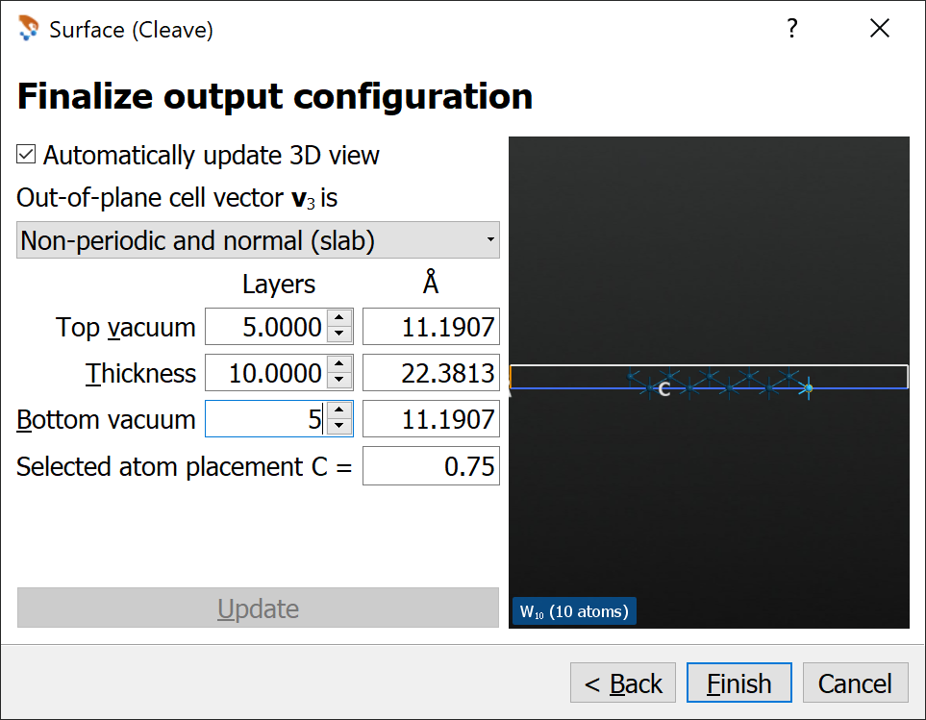• Change the two rightmost W atoms to Fe.
• Rename the structure to W8-Fe2 (press F2).

We can make an initial optimization of the structure using the Quick Optimizer (see image below). Run the optimization, which only takes a few seconds.Send the structure to the Script Generator using the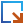icon.

Tip

The Quick Optimizer is an easy way to do an initial optimization of the structure to make it more reasonable before proceeding with the main calculations. It is fast because it uses a ForceField, and will automatically suggest the possibilities from the internal QuantumATK database. However, not all structures will be supported by an appropriate ForceField.

### Structural relaxation¶

Although the structure has been relaxed using the Quick Optimizer, we will still perform a geometry optimization using a DFT calculator. The geometry optimization will be performed using a spin-polarized calculator, while the subsequent analysis will be performed with spin-orbit coupling included.

Now do the following: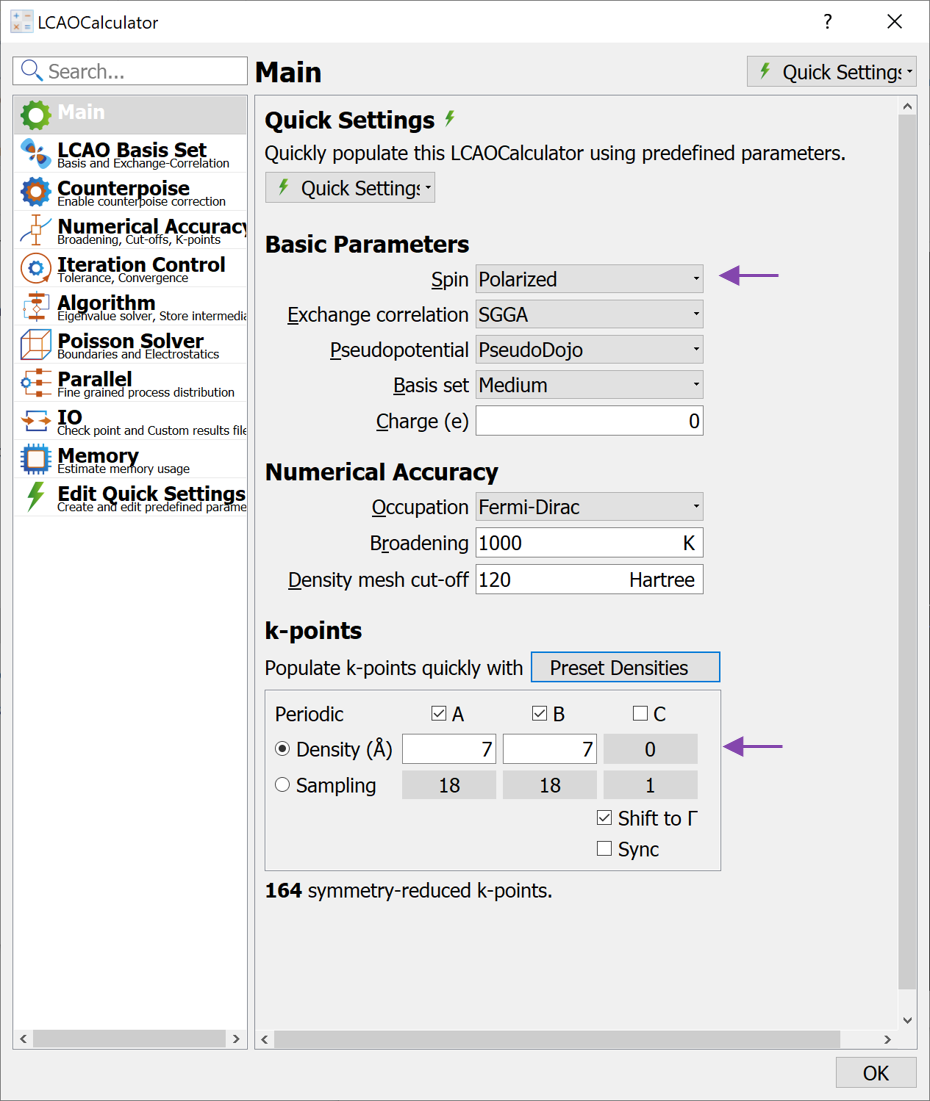• Go to the Poisson Solver page and apply the changes (see image below)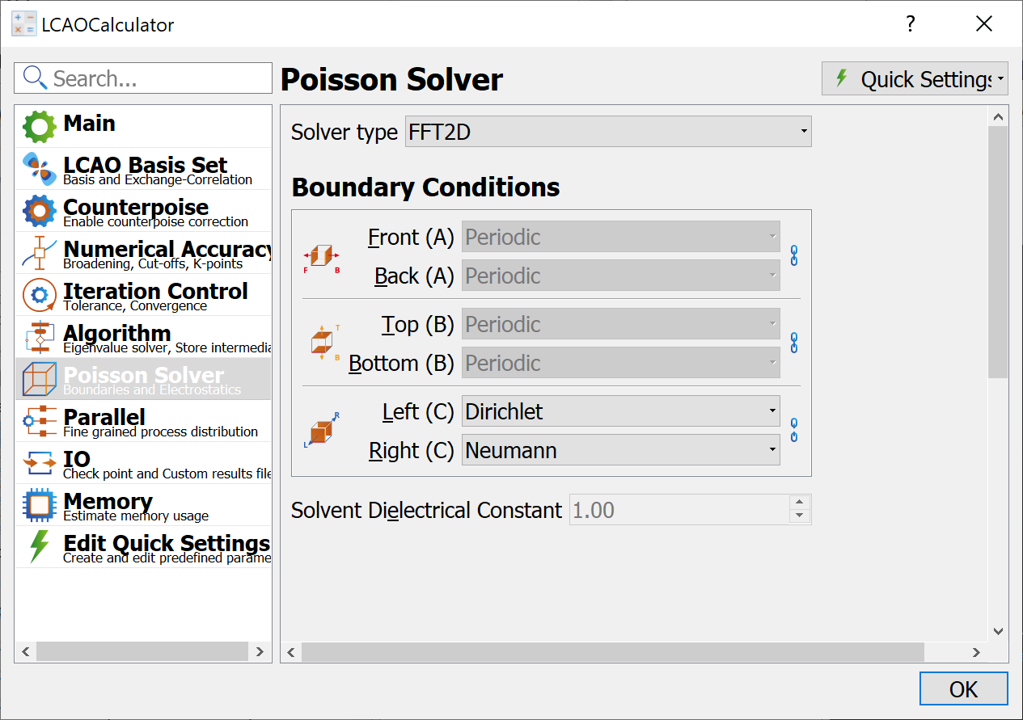• Close the LCAOCalculator widget.

Note

The reason for modifying the Poisson solver boundary conditions is to allow for a dipole shift across the W/Fe interface. At any interface between two materials a certain charge transfer will occur, which will give rise to a surface dipole. Using periodic boundary conditions in the C-direction will result in a electrostatic potential with a certain slope in the vacuum region, effectively acting as an external electric field. By using the combination of Dirichlet and Neumann boundary conditions, the electrostatic potential will be flat in the vacuum regions, but have different values on each side of the slab. Try to add an ElectrostaticPotential or ElectrostaticDifferencePotential analysis to the script and inspect the results after the calculation using the Grid 1D Projector.

Finally change the name of the Results file to W8-Fe2-polarized.hdf5 and run the calculation. The relaxed structure can also be downloaded here W8_Fe2_relaxed_configuration.py.

## Local Density of States calculations¶

Having relaxed the atomic coordinates we are now ready to study the TAMR effect by calculating the local density of states (LDOS). The LDOS contains the DOS at a given energy projected onto a real-space position $$n(E,r)$$.

Send the relaxed configuration to theScript Generator and go through the following steps:

• Add an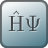LCAOCalculator and open the widget.
• Change Spin to Noncollinear Spin-Orbit
• Increase the k-point density to 7 (select from the Preset Densities).
• Modify the Poisson Solver as described above.
• Close the LCAOCalculator
• Add anInitial State block to the script and open it. In the InitialState widget you can change the initial spin orientation, when changing the Spin Type to Non-collinear (see below). For global spin rotations applied to all atoms the initial spin orientation will usually be kept during the self-consistent calculation. For now you can leave the angles at the default values of $$\theta=0^\circ$$ and $$\phi=0^\circ$$, which corresponds to the out-of-plane magnetization.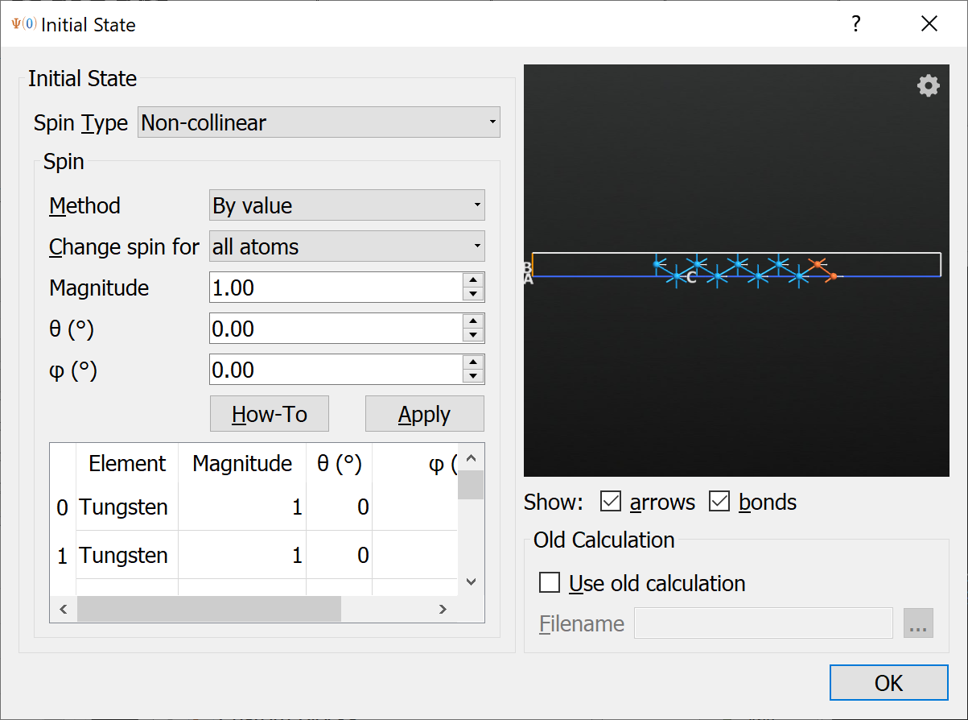Tip

The spin vectors are shown in the 3D window, allowing easy inspection of the initial spin state.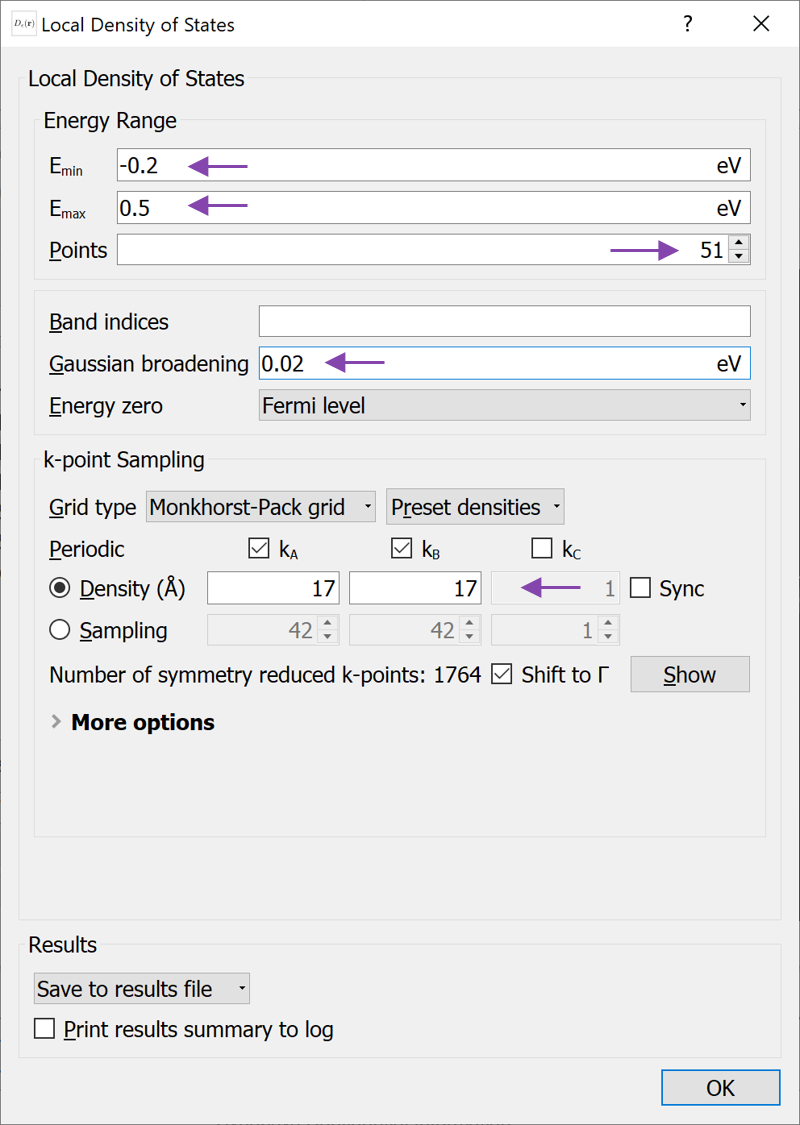Note

LocalDensityOfStates objects can take up a lot of memory, since a real-space grid object is stored for every energy point. In order to reduce the memory of the calculation as well as of the stored .hdf5 file, the number of energy points can be lowered. It is also possible to modify the density of real-space grid points. In order to do the latter, you must send the script to the Editor and add the line density_mesh_cutoff=20*Hartree to the LocalDensityOfStates object as shown below.

# -------------------------------------------------------------
# Local Density Of States
# -------------------------------------------------------------
kpoints = KpointDensity(
density_a=17.0*Angstrom,
density_c=0.0*Angstrom,
force_timereversal=False,
)

local_density_of_states = LocalDensityOfStates(
configuration=bulk_configuration,
kpoints=kpoints,
energies=numpy.linspace(-0.2, 0.5, 51)*eV,
band_indices=All,
energy_zero_parameter=FermiLevel,
)


Now we will change the initial spin to simulate the in-plane magnetization.

• Open theInitial State widget again and change the angles to $$\theta=90^\circ$$ and $$\phi=150^\circ$$, which is the orientation with the lowest energy (try for yourself to scan over $$\phi$$ angles and calculate the total energy at each.) Press Apply to rotate the angles. The spin orientation should now look as below: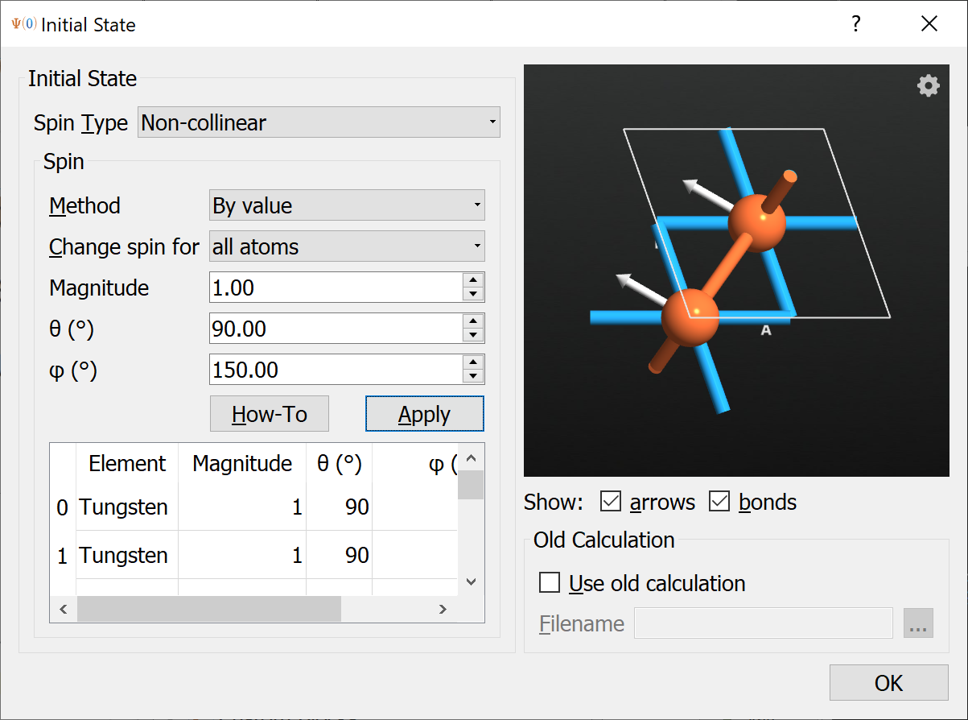Close the InitialState widget and change the Results file to W8-Fe2-theta-90-phi-150.hdf5 and run the calculation.

When both calculations have finished, we are ready to analyze the results.

## Analyzing the results¶

Once the calculation has finished we are ready to analyze the results. Before analyzing the LDOS calculations, take a look at the calculated MullikenPopulations using theViewer. Verify that the spins on the iron atoms are pointing in the expected directions - out of plane (in the z-direction) for the W8-Fe2-theta-0-phi-0.hdf5 file and in-plane for the W8-Fe2-theta-90-phi-150.hdf5 file.

### LDOS analysis¶

Mark theLocalDensityOfStates object in the file W8-Fe2-theta-0-phi-0.hdf5 and open it with the Local DOS Analyzer. The analyzer should appear as shown below. In the STM-like analyzer, you can adjust the tip position using both fractional- and Cartesian coordinates. When the tip position is changed, the LDOS vs. energy plot (upper right) will be updated. Change the tip position to 2 Å above the surface Fe atom at (0, 0, 35.067) Å. Try also to change the energy and see how the contour plot changes. The contour plot in the 3D window with the atomic structure contains the same data as the contour plot in the lower right plot.

Right click on the line in the upper right plot and choose Edit item and change the label Spin Sum to $\perp$, which QuantumATK will automatically parse and show as the symbol.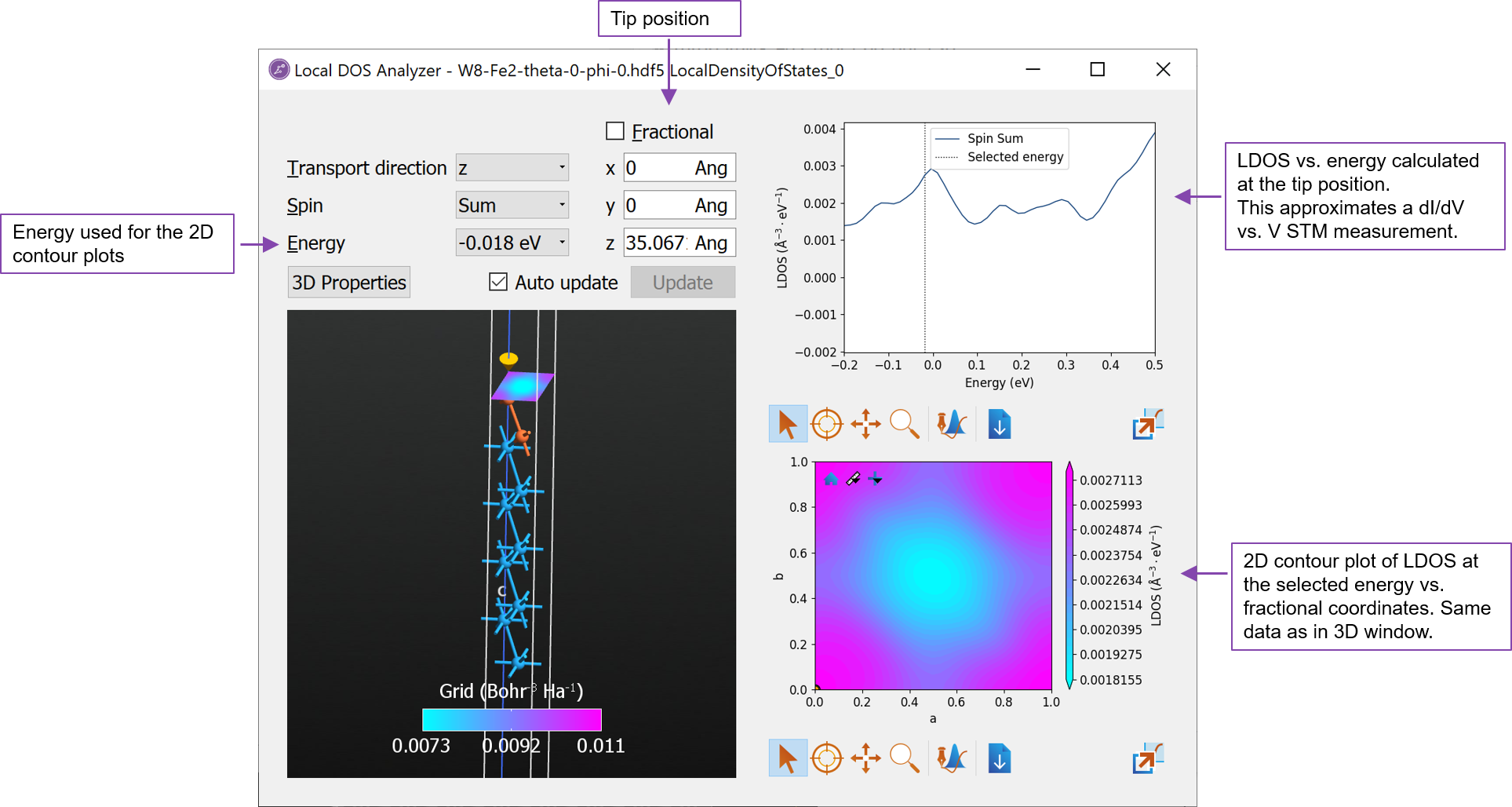Now open the LocalDensityOfStates object from the file W8-Fe2-theta-90-phi-150.hdf5 containing the in-plane magnetization. Apply the same setting for the tip position as above and edit the line in the upper right plot and change the label to $\parallel$. Also change the color and/or line style, such that it is different from the default setting.

Now drag one of the LDOS vs. energy plots onto the other and select Overlay with shared axes to obtain a plot like below. It is evident that the LDOS is different for the two magnetization directions and that there is a TAMR effect.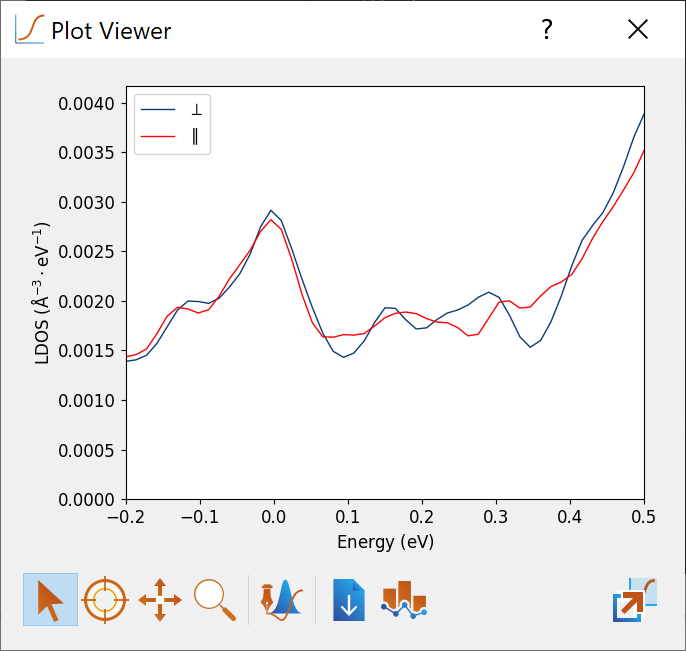In order to quantify the TAMR effect, download and run the script calculateTAMR.py. The script calculates the LDOS vs. energy 2 Å above the surface Fe atom as we just did in the analyzer above. Then it calculates the TAMR using equation (1) above and produces the figure shown below. In qualitative agreement with the experimental findings [NeelSchroderR+13] the TAMR is oscillating as a function of energy (bias voltage in an STM experiment). The magnitude of the calculated TAMR of $$\sim \pm 20 %$$ is also in qualitative agreement with the experimental findings, although the experiments in [NeelSchroderR+13] were obtained for a Co adatom.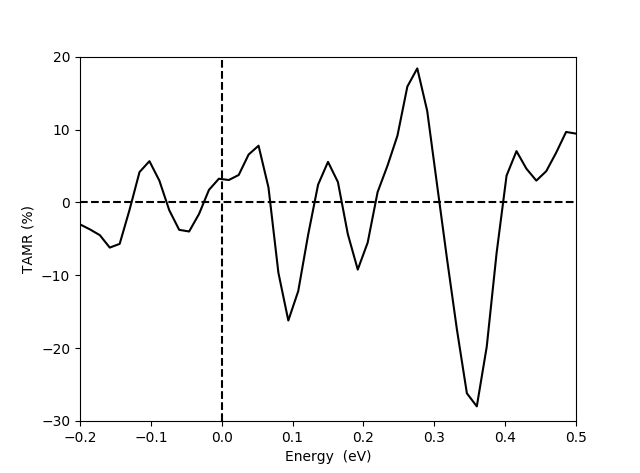In order to get a more direct comparison to the experiments from [NeelSchroderR+13] shown in Fig. 2 at the top, we here present results for a Co adatom positioned on the 2LFe/W(110) slab. The calculations have been performed in the same way as described above. Try for yourself to add a Co atom and relax the structure using the Quick Optimizer in the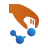Builder by going through the following steps:

• In the Builder, repeat the 2LFe/W(110) structure two times in the A- and B directions.
• Add a Co atom in a bridge position between two surface Fe atoms.
• Relax the configuration using the Quick Optimizer.
• Send the configuration to theScript Generator and setup the spin-orbit and LocalDensityOfStates calculations as described above.

A final script is also given here: W8_Fe2_Co.py. The script loops over the $$(\theta, \phi)$$ angles and performs the self-consistent spin-orbit calculation and LDOS calculation for both angle pairs $$(0,0)$$ and $$(90,150)$$. Run either the scripts you have made yourself or the one given here.

When the calculations are finished (the calculation takes several hours when running on a single node with 16 cores) the results can be analyzed and plotted with the script calculateTAMR-Co-adatom.py to give a figure like the one shown below, on the right. The calculated LDOS and TAMR share many similarities with the experimental results in Fig. 3 (left part):

• The overall shape of the $$dI/dV$$ and LDOS plots agree with a small peak close to the Fermi energy and a larger peak around 0.6 eV.
• Both the calculated and measured TAMR shows a clear dip around the Fermi energy and an oscillating behavior of the TAMR. The oscillation in the calculated TAMR are, however, more rabid than in the experiments.

A potential cause of the differences between experimental and calculated data is the tip distance to the Co adatom. Due to the localized basis set used in the QuantumATK calculations, the LDOS needs to be calculated relatively close to the surface - here 2 Å above the Co atom. It is very likely that the experimental distance is somewhat larger. Also, the calculated results are obtained with a supercell which is a 2x2 repetition of the unit cell. It is likely that this is too small a supercell to match the experimental conditions.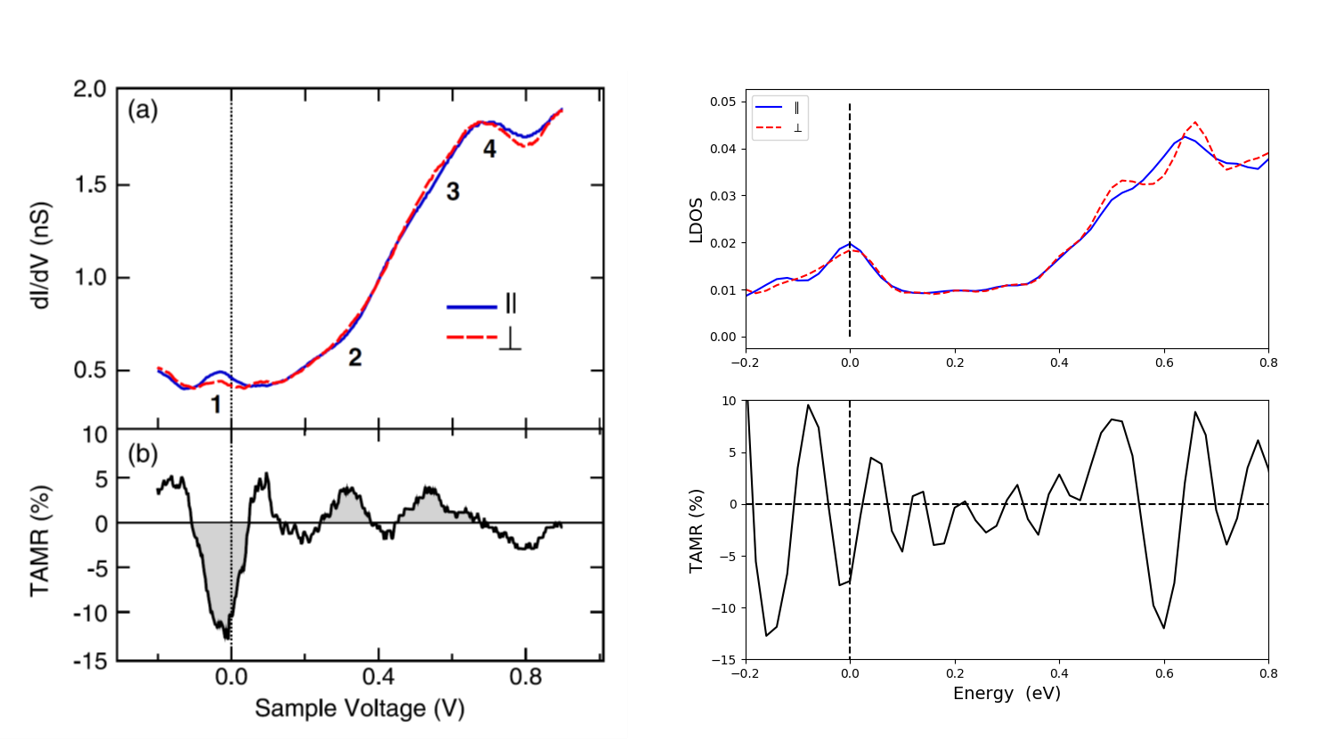Fig. 3 Experimental results (left). (a) $$dI/dV$$ spectra obtained on Co adatoms on domains (red dashed line) and on domain walls (blue solid line) of an Fe double-layer film on W(110). (b) TAMR determined from the spectra in (a). From [NeelSchroderR+13]. The right part of the figure shows the data calculated with QuantumATK.

## COSMICS project¶

TAMR effects are studied in the COSMICS project founded by the European Union’s Horizon 2020 research and innovation program under Grant Agreement No. 766726. More information about the COSMICS project can be found here: http://cosmics-h2020.eu/